# 01背包问题详解

### 01背包问题

01背包问题之另一种风格的描述：#### 暴力解法#### 动态规划#### 1. 吉他行#### 2. 音响行#### 3. 笔记本电脑行#### 4. 等等，再增加一件商品将如何变化呢？3磅容量的最大价值为2000美元！再加上iPhone价值2000美元，总价值为4000美元。新的最大价值诞生了！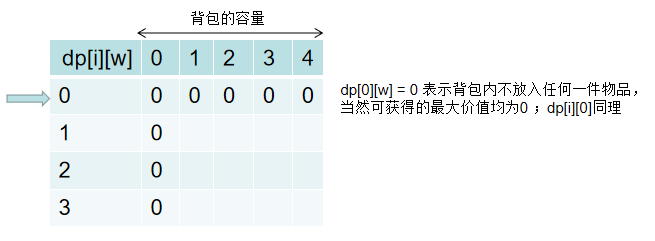• 如果选择它，那么，当前背包的最大价值等于” i 号物品的价值“ 加上 ”减去 i 号物品占用的空间后剩余的背包空间所能存放的最大价值“，即dp[i][k] = value[i] + dp[i-1][k-weight[i]]；

• 如果不选择它，那么，当前背包的价值就等于前 i-1 个物品存放在背包中的最大价值，即 dp[i][k] = dp[i-1][k]

dp[i][k] 的结果取两者的较大值，即：

`dp[i][k] = max(value[i] + dp[i-1][k-weight[i]], dp[i-1][k])`

#### 动态规划

```public class BeiBao01 {
public int maxValue(int[] weight, int[] value, int W) {
//这里假定传入的weight和values数组长度总是一致的
int n = weight.length;
if (n == 0) return 0;

int[][] dp = new int[n + 1][W + 1];
for (int i = 1; i <= n; i++) {
for (int k = 1; k <= W; k++) {
// 存放 i 号物品（前提是放得下这件物品）
int valueWith_i = (k-weight[i-1] >= 0) ? (value[i-1]+dp[i-1][k-weight[i-1]]) : 0;
// 不存放 i 号物品
int valueWithout_i = dp[i - 1][k];
dp[i][k] = Math.max(valueWith_i, valueWithout_i);
}
}

return dp[n][W];
}

public static void main(String[] args) {
BeiBao01 obj = new BeiBao01();
int[] w = {1, 4, 3};
int[] v = {15, 30, 20};
int W = 4;
System.out.println(obj.maxValue(w, v, W));
}
}```

```    public int maxValue(int[] weight, int[] value, int W) {
int n = weight.length;
if (n == 0) return 0;

int[][] dp = new int[n][W + 1];
// 先初始化第 0 行，也就是尝试把 0 号物品放入容量为 k 的背包中
for (int k = 1; k <= W; k++) {
if (k >= weight) dp[k] = value;
else dp[k] = 0; // 这一步其实没必要写，因为dp[][]数组默认就是0
}

for (int i = 1; i < n; i++) {
for (int k = 1; k <= W; k++) {
// 存放 i 号物品（前提是放得下这件物品）
int valueWith_i = (k-weight[i] >= 0) ? (value[i] + dp[i-1][k-weight[i]]) : 0;
// 不存放 i 号物品
int valueWithout_i = dp[i-1][k];
dp[i][k] = Math.max(valueWith_i, valueWithout_i);
}
}

return dp[n-1][W];
}```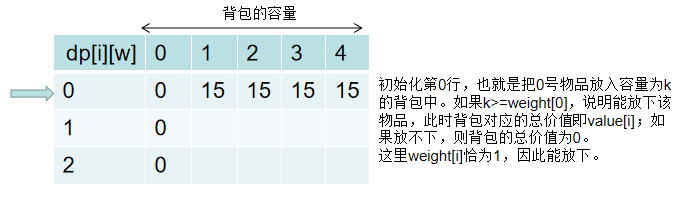（个人更喜欢第二种实现方式，感觉理解起来更友好）

#### 动态规划+压缩空间

```    public int maxValue(int[] weight, int[] value, int W) {
int n = weight.length;
if (n == 0) return 0;
// 辅助空间只需要O(W)即可
int[] dp = new int[W + 1];
for (int i = 0; i < n; i++) {
// 注意这里必须从后向前！！！
for (int k = W; k >= 1; k--) {
int valueWith_i = (k - weight[i] >= 0) ? (dp[k - weight[i]] + value[i]) : 0;
int valueWithout_i = dp[k];
dp[k] = Math.max(valueWith_i, valueWithout_i);
}
}
return dp[W];
}```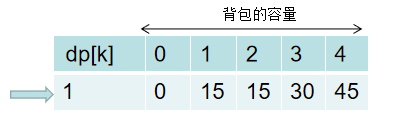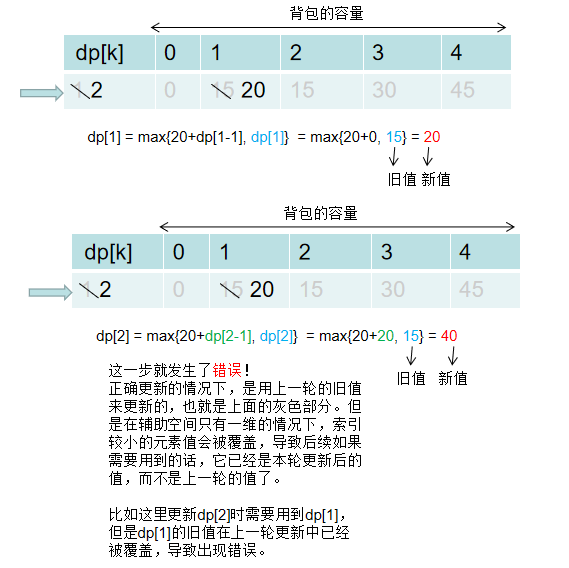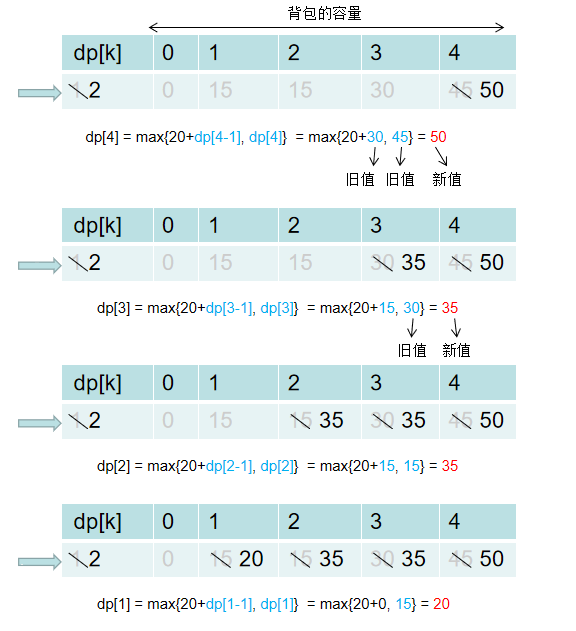```    public int maxValue(int[] weight, int[] value, int W) {
int n = weight.length;
if (n == 0) return 0;

int[] dp = new int[W + 1];
for (int i = 0; i < n; i++) {
//只要确保 k>=weight[i] 即可，而不是 k>=1，从而减少遍历的次数
for (int k = W; k >= weight[i]; k--) {
dp[k] = Math.max(dp[k - weight[i]] + value[i], dp[k]);
}
}
return dp[W];
}```

```for (int k = W; k >= 1; k--) {
int valueWith_i = (k - weight[i] >= 0) ? (dp[k - weight[i]] + value[i]) : 0;
int valueWithout_i = dp[k];
dp[k] = Math.max(valueWith_i, valueWithout_i);
}```

`dp[k] = Math.max(valueWith_i, valueWithout_i) = max(0, dp[k]) = dp[k]`

01背包问题
• ## 01背包问题详解

豆瓜· 今天 09:22:45 · 0浏览
• ## mysql jdbc链接数据库问题

豆瓜· 今天 09:16:34 · 4浏览
• ## 滚动条样式自定义

豆瓜· 今天 09:15:55 · 7浏览

我来说两句